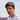# Freehand drawing

July 31, 2016

This post is a part of the series about shapes recognition. This post is also available as a part of a playground.

Naive implementation of rendering user’s freehand drawing is strait forward. We need to listen to touch events from view and construct the path appending lines to each subsequent point.

``````public class LineDrawing: Drawing {
public var canvas: DrawableView?
public var path = UIBezierPath()

public init() {}

public func touchesBegan(at point: CGPoint) {
path.removeAllPoints()
path.moveToPoint(point)
}

public func touchesMoved(to point: CGPoint) {
}

public func touchesEnded(at point: CGPoint) {
}

public func touchesCancelled(at point: CGPoint) {
path.removeAllPoints()
}
}``````

But usually such naive approach does not provide acceptable results. Strokes looks ridgid and angular.

Luckily it’s not very hard to improve our drawing strategy by utilizing Bezier curves. Intead of appending line segments we can append curve segments. We need four points to draw Bezier curve - start point, end point and two control points. So we need to accumulate touch points in array up to four, append curve segment and flush points array.

``````public class CurveDrawing: Drawing {
public var canvas: DrawableView?
public let path = UIBezierPath()
var controlPoints: [CGPoint] = []

public init() {}

public func touchesBegan(at point: CGPoint) {
controlPoints = [point]
path.removeAllPoints()
path.moveToPoint(point)
}

public func touchesMoved(to point: CGPoint) {
guard controlPoints.count == 3 else {
controlPoints.append(point)
return
}
point,
controlPoint1: controlPoints,
controlPoint2: controlPoints
)
controlPoints = [point]
}

public func touchesEnded(at point: CGPoint) {
if controlPoints.count > 1 {
for _ in controlPoints.count..<4 {
touchesMoved(to: point)
}
}
controlPoints = []
}

public func touchesCancelled(at point: CGPoint) {
path.removeAllPoints()
}
}``````

When touchs end we can loose some points at the end of the curve. To avoid that we repeat the last recorded point until we have enouth points to draw the last segment. Dependeing on how many points are missing it can result in a line or curve segment.

Looks better but still not ideal. As you can see there are obtuse angles at the points where two curves connects. We can avoid that by shifting this point to the median of second control point of the first curve and the first control point of the next curve.

Instead of accumulating four control points we will take one more and use it as a first control point of the second curve. Then we calculate new end point for the first curve and create a curve itself. At the end we flush control points array and store there shifted end point (it will be start point if the next curve) and the first control point of the next curve that we already have.

``````public class SmoothDrawing: Drawing {
//The rest of the code is the same as in CurveDrawing

public func touchesMoved(to point: CGPoint) {
guard controlPoints.count == 5 else {
controlPoints.append(point)
return
}
let endPoint = CGPoint(
x: (controlPoints.x + point.x)/2,
y: (controlPoints.y + point.y)/2
)
endPoint,
controlPoint1: controlPoints,
controlPoint2: controlPoints
)
controlPoints = [endPoint, point]
}

public func touchesEnded(at point: CGPoint) {
if controlPoints.count > 1 {
for _ in controlPoints.count..<5 {
touchesMoved(to: point)
}
}
controlPoints = []
}
}``````

Lastly in iOS 9 we have access to coalescing touches (when touch moves we can get more touch points between previous and current touch location), touches prediction and precies touch location. They can be used to achieve even more accurate result. If we have access to coalesced touches we can simply process them one by one as we normally do for other touches. Touch prediction can be used to complete smoothed path in a more intellegent way. Also we can access other touch parameters such as force (to control the path thikness) or stylus orientation. Play with it if you have access to supported devices and check out WWDC session on that topic.Ilya Puchka
iOS developer @Babylon